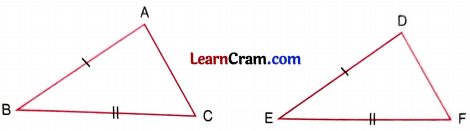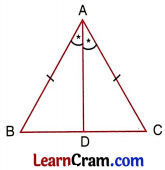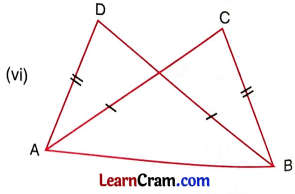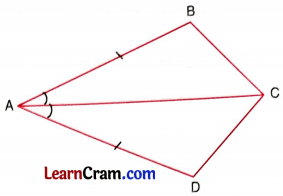# DAV Class 7 Maths Chapter 9 Worksheet 2 Solutions

The DAV Maths Class 7 Solutions and DAV Class 7 Maths Chapter 9 Worksheet 2 Solutions of Construction of Triangles offer comprehensive answers to textbook questions.

## DAV Class 7 Maths Ch 9 WS 2 Solutions

Question 1.
In ∆ ABC and ∆ DEF, AB = DE and BC = EF (See fig.). What additional information is required to make the two triangles congruent by SAS congruence condition ?In ∆ ABC and ∆ DEF,
AB = DE and BC = EF
included ∠ABC = ∠DEF
Hence, additional condition for congruency required is ∠ABC must be equal to

Question 2.
∆ ABC is isosceles with AB = AC (See fig.).Line segment AD bisects ∠A and meets the base BC at D. Find the third pair of corresponding parts which make ∆ ADB ≅ ∆ ADC by SAS congruence condition. Is it true to say that BD = CD? Why?
In ∆ ABD and ∆ ACD,
AB = AC [given]
∆ABD = ∆ ACDQuestion 3.
In the given figure, AB ∥ DC and AB = DC.(i) Is ∠BAC = ∠DCA? Why?
AB ∥ DC and AC is transversal (given)
∴ ∠BAC = ∠ACD (alternate angles)
AB = DC (given)
AC = AC (Common)

(ii) Is ∆ABC ≅ ∆CDA by SAS congruence condition?
∴ ∆ABC ≅ ∆CDA (By SAS)

(iii) State the three facts that you have used to answer (ii).
(a) AB = DC (given)
(b) AC = AC (Common)
(c) ∠BAC = ∠ACD (Alternate angles)

Question 4.
In the given figure, which pairs of triangles are congruent by SAS congruence condition?In ∆ABC and ∆ DEF,
AB = DE = 3 cm (given)
BC = DF = 4 cm (given)
∠ABC = ∠EDF = 70° (given)
∆ABC ≅ ∆DEF (By SAS)In ∆PQS and ∆RQS,
PS = QR = 4 cm (given)
QS = QS = 5.8 cm (given)
∠P = ∠R = 90° (given)
∆PQS ≅ ∆RQS (By RHS)In ∆PQR and ∆XYZ,
PR = XY = 4 cm (given)
QR = YZ = 2 cm (given)
∠PRQ = ∠X YZ = 55° (given)
∆PQR ≅ ∆XYZ (By SSS)In ∆ABD and ∆ABC,
DB = AC (given)
AB = AB (Common)
∆ABD ≅ ∆ABC (By SAS)In ∆PQS and ∆RQS,
PS = QR = 4 cm (given)
QS = QS = 5.8 cm (given)
∠P = ∠R = 90° (given)
∆PQS ≅ ∆RQS (By RHS)In ∆ABD and ∆ABC,
DB = AC (given)
AB = AB (Common)
∆ABD ≅ ∆ABC (Common)Question 5.
In the given figure, AB = AD and ∠BAC = ∠DAC(i) State in symbolic form, the congruence of two triangles ABC and ADC is true. Also, state the congruence condition used.